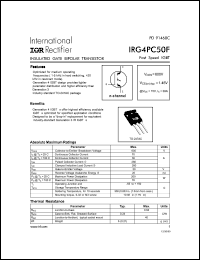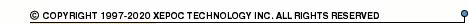More than479 035 registered clientsIRG4PC50 series datasheets. Manufacturer: International Rectifier.

 IRG4PC50 Insulated gate bipolar transistor. VCES = 600V, VCE(on)typ. = 1.45V @ VGE = 15V, IC = 39A in 3-pin TO-247AC package. Operational temperature range from -55°C to 150°C. Datasheet*) IRG4PC50FD Insulated gate bipolar transistor with ultrafast soft recovery diode. VCES = 600V, VCE(on)typ. = 1.45V @ VGE = 15V, IC = 39A in 3-pin TO-247AC package. Operational temperature range from -55°C to 150°C. Datasheet*) IRG4PC50K Insulated gate bipolar transistor. VCES = 600V, VCE(on)typ. = 1.84V @ VGE = 15V, IC = 30A in 3-pin TO-247AC package. Operational temperature range from -55°C to 150°C. Datasheet*) IRG4PC50KD Insulated gate bipolar transistor with ultrafast soft recovery diode. VCES = 600V, VCE(on)typ. = 1.84V @ VGE = 15V, IC = 30A in 3-pin TO-247AC package. Operational temperature range from -55°C to 150°C. Datasheet*) IRG4PC50S Insulated gate bipolar transistor. VCES = 600V, VCE(on)typ. = 1.28V @ VGE = 15V, IC = 41A in 3-pin TO-247AC package. Operational temperature range from -55°C to 150°C. Datasheet*) IRG4PC50U Insulated gate bipolar transistor. VCES = 600V, VCE(on)typ. = 1.65V @ VGE = 15V, IC = 27A in 3-pin TO-247AC package. Operational temperature range from -55°C to 150°C. Datasheet*) IRG4PC50UD Insulated gate bipolar transistor with ultrafast soft recovery diode. VCES = 600V, VCE(on)typ. = 1.65V @ VGE = 15V, IC = 27A in 3-pin TO-247AC package. Operational temperature range from -55°C to 150°C. Datasheet*) IRG4PC50W Insulated gate bipolar transistor. VCES = 600V, VCE(on)typ. = 2.3V @ VGE = 15V, IC = 27A in 3-pin TO-247AC package. Operational temperature range from -55°C to 150°C. Datasheet*) IRG4PC50F INSULATED GATE BIPOLAR TRANSISTOR(Vces=600V, Vce(on)typ.=1.45V, @Vge=15V, Ic=39A) Datasheet*)